Everyday maths 1 (Wales)

Start this free course now. Just create an account and sign in. Enrol and complete the course for a free statement of participation or digital badge if available.

Free course

# 4.4 Decimal problems

Now try the following activity.

## Activity 33: Using decimals

Solve these problems involving decimal numbers without using a calculator.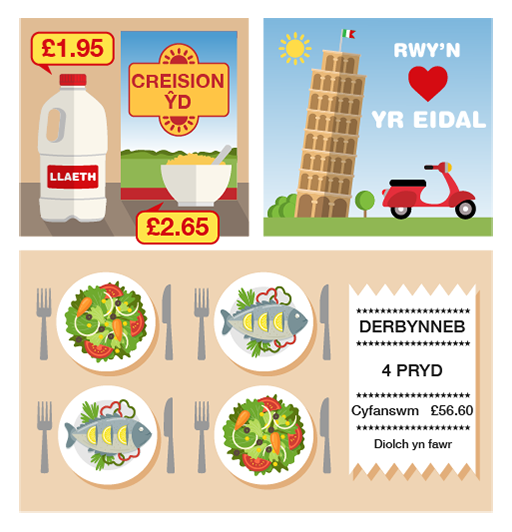Figure 25 Using decimals
1. You buy a box of corn flakes for £2.65 and a bottle of milk for £1.98.
• a.What is the total cost of these items?
• b.You pay for them with a £5 note. How much change should you get?
2. You go on holiday to Italy. The rate of exchange is £1 = €1.4. How many euros do you get for £8?
3. You go out for a meal with three friends, and the total cost of the meal is £56.60. You decide to split the bill equally. How much does each of you pay?
4. Convert 6.25 m to cm. (Remember that 100 cm = 1 m.)

1. The answers are as follows:
• a.Add the cost of the two items: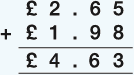(Keep the decimal points in line.)

The total cost of the items is £4.63.

• b.Take away the total cost from £5: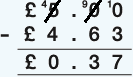You should get 37p change from £5. You may have used a different method to work this out.

2. Multiply the exchange rate in euros (€1.4) by the amount in pounds (£8):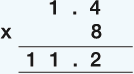So £8 = €11.20. You may have used a different method to work this out.

3. Divide the total cost (£56.60) by the number of people (4):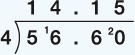You would each pay £14.15.

4. To convert 6.25 m into cm, you need to multiply the amount by 100.

• 6.25 × 100 = 625

So the answer is 625 cm.

## Summary

In this section you have learned about how:

• the value of a digit depends on its position in a decimal number
• to approximate answers to calculations involving decimal numbers
• to add, subtract, multiply and divide using decimal numbers.

This will help when working with money and measurements.

FSM_1_CYMRU#### Live in Wales? Free your ambition with a paid part-time course with the OU in Wales.

With grants of up to £4,500* to help with living costs, and tuition fee loans to cover course fees,
now's the time to take that next step.

#### Find out more

*Eligibility rules apply for financial support.